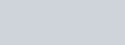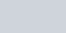Stay Update with us

Email Subscription for Get Latest Notification and MCQ Quiz

# 2011 CTET Paper-1 Question with Answer

2011 CTET Paper-1 Question with Answer

 Child Development Mathematics Environment studies Language-I(Eng) Language-II(Hindi)

#### MATHEMATICS

Directions: Answer the following questions by selecting the most appropriate option.

31. While teaching a comparison of fractions which the numerators are the same e.g. 3/5 and 3/7 Rohit’s response was “since the numerators are same and since 7 is larger than 5, therefore 3/7 is bigger than  3/5 .” This suggests that

(a) Rohit does not know the concept of equivalent fractions

(b) Rohit has not practiced well

(c) Rohit does not understand the magnitude of fractions

(d) Rohit does not know the concept of numerator and denominator

(a) Rohit does not know the concept of equivalent fractions

32. Examine the following matchstick patterns:If the pattern continues, how many matchsticks are needed in the 15th stage?

(a) 61

(b) 62

(c) 105

(d) 65

33. To introduce the concept of fractions, a teacher can begin with

(a) writing fractions in the form of a/b where b ≠ 0

(b) identifying fractional parts of things around them

(c) identifying numerators and denominators of different fractions

(d) finding fractions on a number line

Answer: (a) writing fractions in the form of a/b where b ≠ 0

34.is 3/4 of a ‘unit’. What will be $1\frac{1}{2}$?Answer: (d)35. The number 49532 rounded off to the nearest thousand is

(a) 41000

(b) 50000

(c) 49000

(d) 49500

36. In the following, which is the greatest number?

(a) [(2+2)2]2

(b) (2+2+2)2

(c) (d)2

(d) (2×2×2)2

37. “Start a discussion in the class on things in the child’s environment which roll and slide. Help children to look at their shapes and see how some things roll and others slide.”Suggestions like this have been given in the NCERT textbook of Class-II to help a teacher understand that

(a) discussions supplemented with demonstration help students to understand concepts better

(b) discussions bring multiple perspectives into the classroom

(c) discussion is the best strategy for the mathematics classroom

(d) it is imperative for the teachers to draw the children’s attention to the things around them

Answer: (a) discussions supplemented with demonstration help students to understand concepts better

38. The chapters in the NCERT textbook of mathematics of Class-IV have titles like 38. “The Junk Seller”, “Trip to Bhopal”, “The Way the World Looks”. This shift has been done to

(a) make it interesting by relating it to everyday life

(b) know about selling junk and traveling

(c) challenge the students to guess the mathematical content in the chapters

(d) make them understand differently

Answer: (a) make it interesting by relating it to everyday life

39. The weight of some mangoes is 2 kg 600 g and that of some apples is 1 kg 450 g. The weight of the mangoes is greater than that of the apples by

(a) 1 kg 200 g

(b) 150 g

(c) 4 kg 50 g

(d) 1 kg 150 g

Answer: (d) 1 kg 150 g

40.”Problem solving” as a strategy of doing mathematics involves

(a) activity based approach

(b) estimation

(c) extensive practice

(d) using clues to arrive at a solution

41. Sequence the following tasks as they would be taken up while. developing the understanding of shapes and space across primary classes :

a. Matches the properties of 2-D shapes by observing their sides and corners

b. Describes intuitively the properties of 2-D shapes

c. Sorts 2-D shapes

d. Describes the various 2-D shapes by counting their sides, corners, and diagonals

(a) a, d, b, c

(b) c, a, d, b

(c) d, b, a, c

(d) c, b, d, a

Answer: (b) c, a, d, b

42. If an operator ⬲ is defined as

4 ⬲ 3 = ᷿4 + 5 + 6

5 ⬲ 4 = 5 + 6 + 7 + 8

6 ⬲ 4 = 6 + 7 + 8 + 9

what will  n ⬲ 8 be equal to ?

(a) 8n + 36

(b) n + 36

(c) n + 28

(d) 8n + 28

43. A teacher asked in a class to represent 1/8 of. Which among the following is an incorrect representation?Answer: (c)44. The purpose of a diagnostic test in mathematics is

(a) to fill the progress report

(b) to plan the question paper for the end-term examination

(c) to know the gaps in children’s understanding

(d) to give feedback to the parents

Answer: (c) to know the gaps in children’s understanding

(a) Forty thousand nine hundred twenty-eight

(b) Four lakh seven thousand nine hundred twenty-eight

(c) Four lakh seventy-nine thousand twenty-eight

(d) Forty-seven thousand nine hundred twenty-eight

Answer: (b) Four lakh seven thousand nine hundred twenty eight

46. The length of a rectangle is ‘l’ and its width is half of its length. What will be the perimeter of the rectangle if the length is doubled keeping the width the same?

(a) 6l

(b) 3l

(c) 4l

(d) 5l

47. Which is true for a hexagonal pyramid?

(a) It has two hexagonal faces and six rectangular faces

(b) It has six hexagonal faces joined by six rectangular faces

(c) It has six faces and each face is a hexagon

(d) It has a hexagonal base with six triangular faces meeting at a point

Answer: (d) It has a hexagonal base with six triangular faces meeting at a point

48. How many 4-digit numbers are there in the Hindu-Arabic Numeration System?

(a) 9999

(b) 9000

(c) 99

(d) 8999

49. Vikas teaches mathematics to a class of 56 students. He believes that conducting a test is effective if the feedback is given immediately. He conducted a short class test of 10 marks. What is the best possible way of giving feedback effectively?

(a) He can have a whole class discussion on ways in which they have got their solutions and which is the effective strategy to arrive at the correct answer

(b) Pick out any copy at random and discuss the method followed in the copy on the board

(c) He can let the students check each other’s answer

(d) He can explain the solution of each problem on the board and ask the students to check their answer on their own

Answer: (a) He can have a whole class discussion on ways in which they have got their solutions and which is the effective strategy to arrive at the correct answer

50. When teaching ‘shapes’, a teacher can plan a trip of historical places as

(a) field trips have been recommended by CBSE, so they are a must

(b) shapes are an integral part of any architecture and such trips encourage connections across disciplines

(c) she has completed most of the syllabus well in time and needs to provide leisure

(d) it would be a good break from the routine mathematics class and an opportunity to improve communicative skills

(b) shapes are an integral part of any architecture and such trips encourage connections across disciplines

51. In a dice, the numbers on the opposite faces add up to 7. Which among the following will fold into a dice?Answer: (c)52. To introduce the concept of area, a teacher can start with
(a) calculating the area of figures with the help of counting unit square
(b) explaining of formulae for finding the area of figures of different shapes
(c) comparing the area of any figure with the help of different objects like palm, leaf, pencil, notebook, etc.
(d) calculating area of a rectangle by finding length and breadth of a rectangle and using the formula for the area of a rectangle (i.e. length x breadth)
Answer: (c) comparing area of any figure with the help of different objects like palm, leaf, pencil, notebook, etc.
53. When faced with word problems, Rajan usually asks “Should I add or subtract?” “Should I multiply or divide?”. Such questions suggest
(a) Rajan lacks understanding of number operations
(b) Rajan cannot add and multiply
(c) Rajan seeks opportunities to disturb the class
(d) Rajan has problems in comprehending language
54. A rhombus has diagonals of length 8 cm and 6 cm. Find its perimeter.
(a) 24 cm
(b) 28 cm
(c) 18 cm
(d) 20 cm
55. Look at the following table:Which bus takes the least time to reach Mathura from New Delhi?
(a) Bus 3
(b) Both Bus 2 and Bus 3 take equal time
(c) Bus 1
(d) Bus 2
56. When teaching the addition of fractions, a teacher came across the following error: 1/2 + 1/3 = 2/5 What remedial action can the teacher take in such a situation?
(a) Help the child to understand the magnitude of each fraction
(b) Help the child to understand the concept of LCM
(c) Ask the child to practice as much as she can
(d) No intervention is needed because she will understand as she grows
Answer: (b) Help the child to understand the concept of LCM
57. Sequence the following tasks as they are taken up while developing the concept of measurement :
a. Learners use standard units to measure length.
b. Learners use non-standard units to measure length.
c. Learners verify objects using simple observation
d. Learners understand the relationship between metric units.
(a) c, b, a, d
(b) d, a, c, b
(c) a, b, d, c
(d) b, a, c, d
(a) c, b, a, d
58. The NCF (2005) considers that Mathematics involves ‘a certain way of thinking and reasoning’. From the statements given below, pick out one which does not reflect the above principle:
(a) The method by which it is taught
(b) Giving students set formulae to solve the numerical questions
(c) The way the material presented in the textbooks is written
(d) The activities and exercises were chosen for the class
Answer: (b) Giving students set formulae to solve the numerical questions
59.“These days prices have started rising.” Which among the following graphs represents this situation?Answer: (a)60. To be a “good” mathematician one must be able to

(a) understand, apply and make connections across the concepts

(b) master the techniques of answering questions

(c) memories most of the formulae

(d) solve the problem in no time

Answer: (a) understand, apply and make connections across the concepts
Pages ( 2 of 5 ): « Previous1 2 3 ... 5Next »

### Quiz-5: Social Studies and Pedagogy MCQ Questions with Answer

error: Content is protected !!